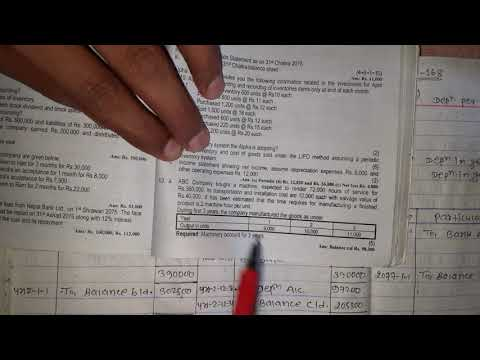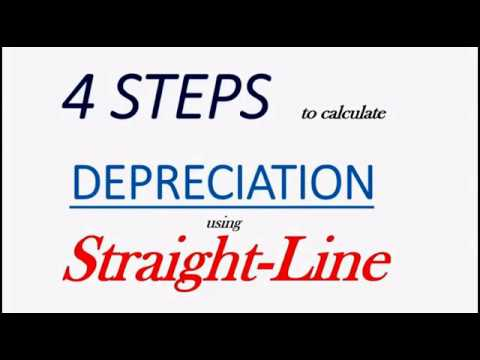# Activity Method of Depreciation Example LimitationLet us understand the units-of-activity method of depreciation by taking an example. This method can be contrasted with time-based measures of depreciation such as straight-line or accelerated methods. Momhil Inc. bought a professional coffee machine costing \$100,000. Over its useful life it is estimated that it can process 5,000 kgs of coffee or 200,000 servings.

Different from Straight-line depreciation, asset useful life will express as the expected units, and the expense charged to the income statement will depend on the number of units produce within the period. This depreciation method will rely on the actual usage of assets so it will be more accurate than other methods. Unit of production method calculates depreciation charge on the basis of actual usage of asset. The expected total output, usually express in units produced or hours worked, is estimated at the time of acquisition and based on the activity in the period proportionate depreciation is calculated. Although the right name of method is unit of production but units of production is widely used. Under activity method, the depreciation expense is calculated on the basis of asset’s actual operational activity such as the number of units produced or the number of hours the asset has used during the period.

## Activity Method Depreciation

The unit of production method is a method of calculating the depreciation of the value of an asset over time. It becomes useful when an asset’s value is more closely related to the number of units it produces rather than the number of years it is in use. This method often results in greater deductions being taken for depreciation in years when the asset is heavily used, which can then offset periods when the equipment experiences less use.

• Straight-line method calculates depreciation on the basis of time and asset is depreciated even if it is idle.
• Then, multiply that quotient by the number of units (U) used during the current year.
• The activity depreciation method is a cost accounting technique that changes the cost behavior with the fluctuating output.
• “Unit of production” means the unit entity uses to measure its production which can be liters, kilograms, miles, hours, production runs and number of units produced.
• The other type of depreciation such as straight line and declining is depending on the time.

Depreciation expense for a given year is calculated by dividing the original cost of the equipment less its salvage value, by the expected number of units the asset should produce given its useful life. Then, multiply that quotient by the number of units (U) used during the current year. Although the right phrase is “unit of production” but accounting world have accepted it as “units of production”. Activity method is excellent in matching expense with revenue in situations where service efficiency of the asset is decreased by its use.

## Unit of Production Method of Depreciation

The modified accelerated cost recovery system (MACRS) is a standard way to depreciate assets for tax purposes. The unit of production method most accurately measures depreciation for assets where the “wear and tear” is based on how much they have produced, such as manufacturing or processing equipment. The activity depreciation method is a cost accounting technique that changes the cost behavior with the fluctuating output. This means that the costs are assigned to the activities based on their usage or consumption. The activity depreciation method is used to allocate the depreciation expense base on the production activity.Activities Based Depreciation allows the management to match between revenue and depreciation expense. It is easy to prepare a budget and project net profit during the period. It would be hard to apply this method to depreciate office buildings or other assets that are not linked with the production unit. You purchase a construction vehicle for your business for \$225,000 and you expect it to have a life of 15,000 hours with a salvage value of \$5,000 after about 10 years.

## Feature of Activity-Based Depreciation

In the high season, the production increase as well as the depreciation expense. The unit-of-activity method is a little different method for calculating depreciation. What it does is, it takes a total number of units a plant or asset is expected to produce during its lifetime for the depreciation purpose. “Unit of production” means the unit entity uses to measure its production which can be liters, kilograms, miles, hours, production runs and number of units produced. Entity can measure its production or output in any unit it deems fit and is not restricted only to units obtained as a result of production activity.

This method is designed to better match the costs with the revenue generated by the output. In other words, it ensures that the costs are properly assigned to the activity that caused them. We can calculate the activity method of deprecation by estimating the total output in the lifetime of the asset. And then calculate the cost per unit of output which is simply the purchase price less scrap value and divided by total output. Then we can get the depreciation expense per year by multiplying the output during the year with the cost per unit of output. Instead, the depreciation is expressed and calculated based on the asset’s usage.

## Why Should We Use Activity-Based Depreciation?

In the first year of purchase, this Excavator has used for 1,500 hours. Company ABC purchases a new Excavator that cost \$ 220,000 for a construction project. The Southern company uses a delivery truck whose cost is \$50,000 and the salvage value is zero. The company plans to retire the truck after it has been driven 250,000 miles. You purchase a car for your business for \$22,000 and you expect it to have a life of 60,000 miles with a final salvage value of \$2,000.

## Activity-Based Vs Other Depreciation Methods

In other words, this method focuses on the real use of the asset in production process rather than just the passage of time. Other names used for activity method of depreciation are variable charge approach and units of output method. Units of production method or allocates cost of asset on the basis of actual use of asset and thus matching the cost and benefits in a much better way than any other depreciation method. Straight-line method calculates depreciation on the basis of time and asset is depreciated even if it is idle. On the other hand declining balance method applies a consistent depreciation rate that may be more or less than actual wear and tear of asset.

## What Does the Unit of Production Method Tell You?

This method is suitable for companies that desire high depreciation during the years of high productivity and low depreciation during the years of low productivity. The company will record a depreciation expenses of \$14,000 for the year 2022. The wheel loader will be fully depreciated after completing 15,000 hours of work – that is its productive life.